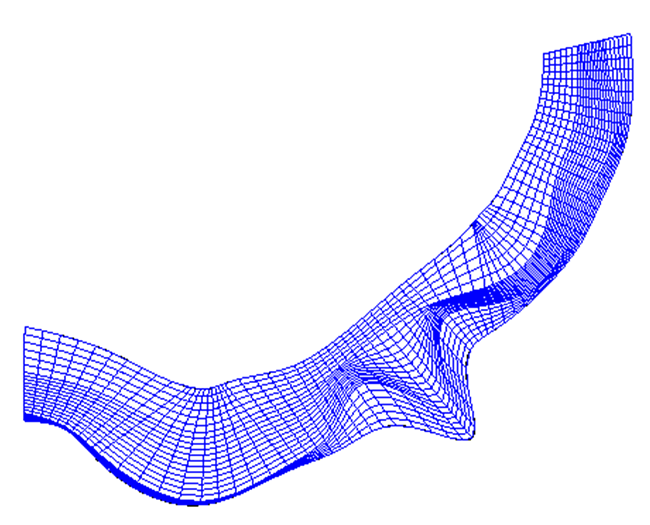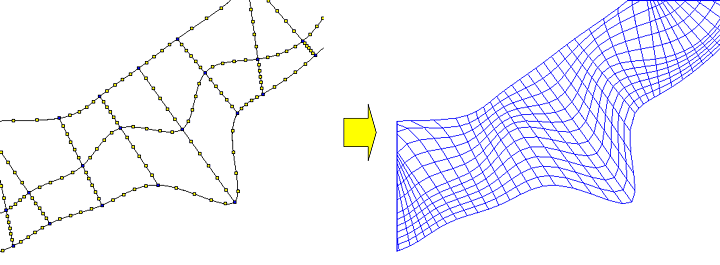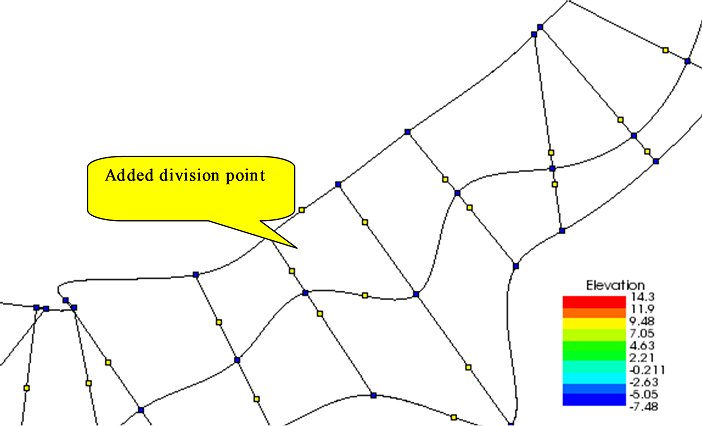# [Create grid from Cross-Section Data] (2-D structured grid)¶

Description: Creates a grid from Cross-Section Data. In addition to transverse lines being set, division points are set on the transverse lines, the river centerline and left/right bank lines. Figure 263 shows an example of a grid created by this algorithm.

Before using this algorithm, you need to import Cross-Section Data.Figure 263 Example of a grid created from Cross-Section Data

The following lines of Cross-Section Data are used as grid lines. In addition, division points added by the user are used to create a grid.

• River transverse line
• River centerline: the curve made by linking the center point of each river transverse line
• Left bank end line: the curve made by linking the left bank end of each river transverse line
• Right bank end line: the curve made by linking the right bank end of each river transverse line

Figure 264 shows an example of grid division points and the grid made from these division points.Figure 264 Example of grid division points and the grid made from these division points

Select [Grid Creation] from the menu. The [Grid Creation] dialog (Figure 265) will open. Specify the region where the grid is to be made and click on [OK]. The grid is generated according to the division points. Operations related to the division points are done from the menu shown in Menu items.

## [Add Division Points] (A)¶

Description: Adds division points to the selected line.

Select the line to which you want to add division points (e.g., river centerline, left bank end line, right bank end line, river transverse line) by clicking on it. Figure 266 shows an example of a display after selecting a line. Then, select [Add Division Points] from the menu. The [Add Division Point] dialog (Figure 267) will open. Input number of divisions and select division method, and click on [OK]. Division points are added to the line (Figure 268).Figure 268 Example of a display after division points have been added

## [Add Division Points Regionally] (R)¶

Description: Simultaneously adds division points to the river centerline and left/right bank lines.

The [Add Division Points Regionally] dialog (Figure 269) will open. Specify the region where division points are to be added and number of divisions, and click on [OK].

## [Delete Division Points] (D)¶

Description: Deletes the selected division points.

Figure 270 shows an example.

## [Move Division Points] (M)¶

Description: Moves the selected division points. This is possible when selecting continuous division points on the same line.

When you select [Move Division Points], the [Move Division Points] dialog (Figure 271) will open. Set [Transfer Quantity] and click on [OK].

## [Reposition Division Points] (P)¶

Description: Relocates the selected division points. This is possible when selecting continuous division points on the same line.

When this is selected, the [Reposition Division Points] dialog (Figure 272) will open. Edit the coordinates and click on [OK].

## Reset to default (R)¶

Description: Discard the modifications of grid creating condition, and restore to the state just after selecting the grid creating algorithm.